# Machine Learning for Zombies

Neural Networks in Bite-Sized Chunks — The Beginning

Neural networks, a.k.a. Multilayer Perceptrons (MLP), are complex algorithms that take a lot of compute power and a *ton* of data in order to produce satisfactory results in reasonable timeframes. That said, when implemented correctly and given enough of the right kinds of data, they can produce results that no other machine learning technique has so far been able to match (with a nod to Gradient Boosted Trees for tabular data…)

# You’ve Seen This Movie Before

So what are they?

Let’s start with what they’re not: neural networks, despite the name and every blog post and intro to machine learning text book you’ve probably read up till now, are not analogs of the human brain. Sorry. There are some *very* surface-level similarities, but the actual functionality of a neural network has almost nothing in common with the neurons that make up the approximately three pounds of meat that sits between your ears and defines everything you do and how you experience reality.

At a high level, they’re just like any other machine learning algorithm:

Just like a lot of other machine learning algorithms, they use the formula “label equals weight times data value plus offset” (or y = w*x + b) to define where they draw their lines/hyperplanes for making predictions. (Note: you may be more familiar with y = mx + b, where m is defined as the slope of the line. In machine learning, that slope is called a weight.) And just like a lot of other machine learning algorithms, they use gradient descent to find the optimal values for w and b in order to match the training label y most closely across their entire data set. If you already understand linear classifiers like the Perceptron and logistic regression, you’ve got a great start on the concepts for neural networks.

Neural networks and logistic regression have some other things in common as well. Logistic regression starts off with an assumption about the shape of the training labels (the Sigmoid function). Neural networks also make use of an assumption about the labels as well. Sigmoid is one of the options, but you can also use Softplus (a curved line, continuous value) or ReLU (which uses a hinge formula — similar in nature to the hinge loss used by Support Vector Machines — of either 0 or some positive value). In practice today, ReLU , or Rectified Linear Unit, is usually the preferred approach, so I will use that as my assumption about the labels as I write.

## …But It’s Not Exactly the Same…

One of the first differences between neural networks and other machine learning algorithms is the initial assumption about weights. Whereas most machine learning algorithms start with a weight assumption of 0 and modifies from there, neural networks start with non-zero weights that are typically randomly generated. For linear classifiers that are looking for a straight line or hyperplane, or if you’re assuming your labels form a convex shape, it really doesn’t matter what the initial weights are. Starting from 0 is just an easy default. However, if you’re assuming squiggly lines instead of straight ones, you don’t always want to start from the same location. In fact, you may want to run the algorithm multiple times, using different initial weight conditions, to determine where the best predictive power you can find exists. The bias (offset, or b) values for neural networks can start off as 0, but the weights you use to multiply against x need to be something else.

Another difference between neural networks and logistic regression is what they do with that assumed label distribution. With logistic regression, the Sigmoid function is kind of the stopping point — it’s all downhill from there (in case you missed it, that was a gradient descent joke.) However, in neural networks, the assumption is called an Activation Function. It is used to generate some values based on your data, but then these values are used as an input to another step in the process.

# Terminology and Diagrams

The various stages of neural network data processing are called “nodes.” You have an input node, which is where your data starts, and an output node, which is where the prediction ends up. In addition, the activation layer in the middle is also considered a node. These nodes are connected by the math formulas we apply (w*x + b) to either the original data or to the result of the activation node function on the original data — denoted as f(x) below: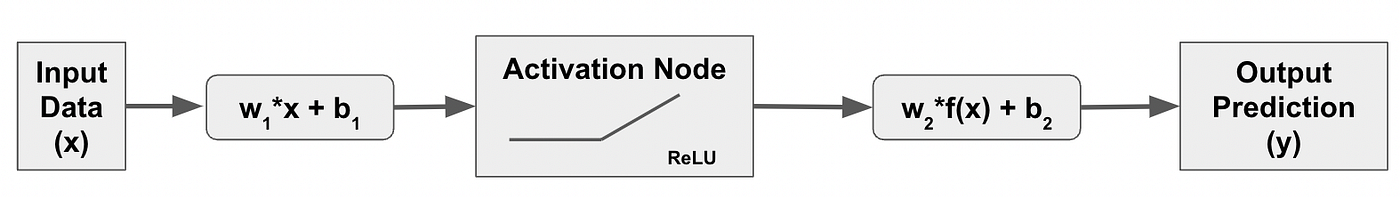The core of a neural network. My zombie is starting to take notice.

The activation node is referred to as a “hidden layer.” You can have more than one of these hidden layers, and why you might want to do that will be a topic for another post. The image above is often referred to as a “neuron” (again, in part due to the surface-level similarity…) It isn’t actually finished though. In order to be a “network” you have to be connected to something, and it turns out that neural networks at a minimum require two neurons. So you might think that a neural network might look like this:

But in reality, we simplify the last stage a bit by summing up all of the w*x values and add just a single offset to get our prediction, so the real neural network would be this:

# What They Do

In future posts we’ll actually create some data and run through some calculations, but for this post, let’s assume we’ve already done all of this and we end up with a couple of lines that look like this (note: negative weights will flip the direction of the line). The blue line represents the standalone results of the first neuron, and the red line represents the standalone output of the second neuron. The y-axis values are the predictions for each neuron for a given value of x:

These lines were the result of using the ReLU activation function and the random values for weights and offset, and we’re pretending we didn’t do the simplification at the end for illustration purposes. Notice that the two lines start at different offset values (blue +5, red -1), which represent the default predictions for the neuron until the ReLU activation kicks in. The ReLU activation means all predicted values will stay at those offsets until the the x value hits a certain point. In the case of the blue line and due to the random weights chosen, this activation results in a slightly postive prediction slope that begins when x = 5. For the red line, the values are 0 until x = 14, at which point the predictions sharply slope negative.

Now to get to the “final” output (for now) we need to add these two lines together. So our final offset value is going to be the midpoint between +5 and -1, or 2.5, which becomes our predicted value until we get to a hinge point. The slope of both lines is 0 until x = 5, so this does not change. When x = 5, only the blue line has any effect (the red line slope is being added but still equals zero) so between 5 and 14 the slope of the prediction function exactly matches the blue line. However, when x = 14, the sharp negative prediction slope of the red line gets added to the light positive prediction slope of the blue line, resulting in a moderate negative prediction slope from x = 14 and beyond. The function would visualize something like this:

So at a very high level, that’s what a neural network is and what it initially does. We still have a lot of ground to cover in terms of the actual math for this “forwards propogation” through the neural network nodes, including adding in additional input nodes, hidden layers, and multiple outputs. We also need to cover how the neural network uses gradient descent and the chain rule to “backwards propogate” and find the best weights for each neuron to minimize training loss. Sizing your neural network — selecting number of neurons and hidden layers and nodes per hidden layer — will be an important consideration. And then overfitting — oh my gosh is this approach **amazing** at overfitting your training data — will need to be addressed as well. And this is just so that we can get through what is arguably a relatively simple implementation of a neural network. So saddle up, buckle in, or whatever your metaphor of choice might be. This is going to be a ride.

--

--

--

## More from MLearning.ai

Data Scientists must think like an artist when finding a solution when creating a piece of code. ⚪️ Artists enjoy working on interesting problems, even if there is no obvious answer ⚪️ linktr.ee/mlearning 🔵 Follow to join our 18K+ Unique DAILY Readers 🟠

## Machine Learning Must-Know## Backpropagation step by step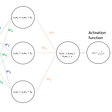## Converting Tensorflow Model to Tensorflow Lite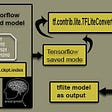## Boosting and Bagging: How To Develop A Robust Machine Learning Algorithm## My Learning Path into Artificial Intelligence and Machine Learning -(Part 1)## Relu Activation Function(ReLU)## Resources for Machine Learning: From the basics to working models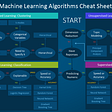## ICU Survival Prediction using Ensemble Learning (Stacking )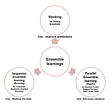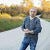## Jason Eden

Data Science & Cloud nerd with a passion for making complex topics easier to understand. All writings and associated errors are my own doing, not work-related.

## More from Medium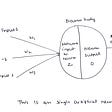## Uncovering the Deep State… of Neural Networks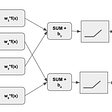## Applications of Deep Learning in Health Informatics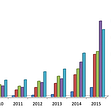## My Writing Plan for 2022